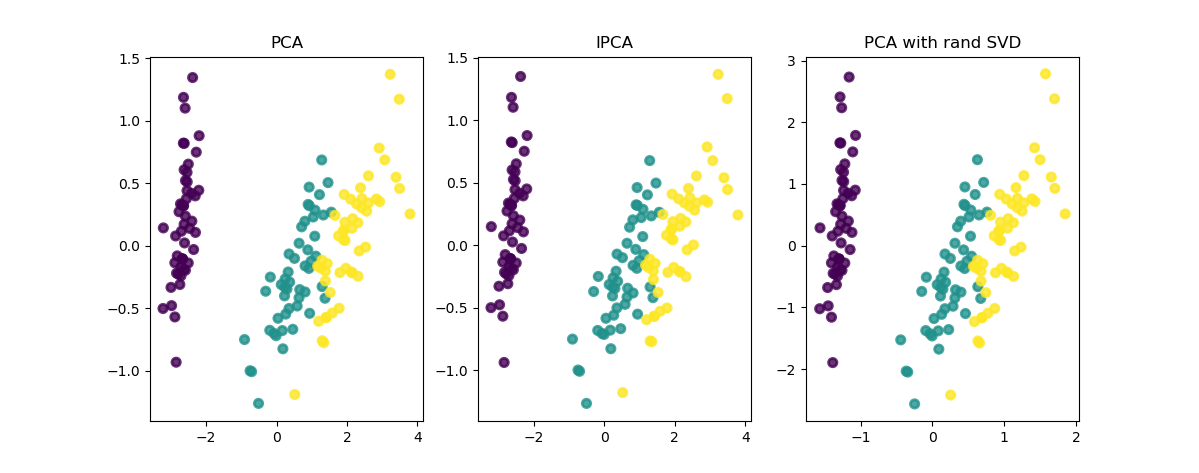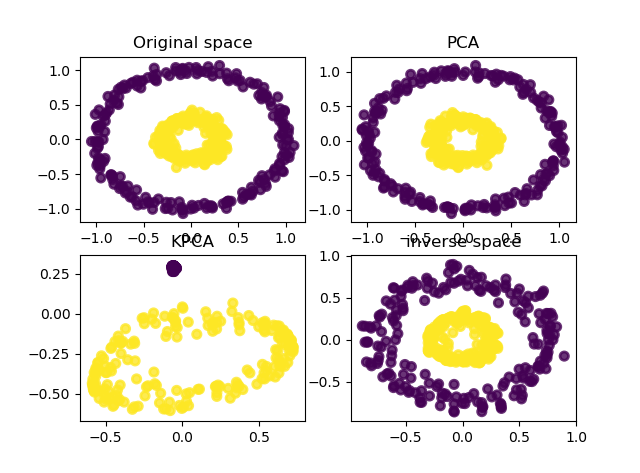# python机器学习库sklearn——降维

python数据挖掘系列教程

## PCA主成分分析

PCA 用于对一组连续正交分量中的多变量数据集进行方差最大方向的分解。 在 scikit-learn 中， PCA 被实现为一个变换对象， 通过 fit 方法可以降维成 n 个成分， 并且可以将新的数据投影(project, 亦可理解为分解)到这些成分中。

PCA 对象非常有用, 但对大型数据集有一定的限制。 最大的限制是 PCA 仅支持批处理，这意味着所有要处理的数据必须适合主内存。 IncrementalPCA 对象使用不同的处理形式使之允许部分计算， 这一形式几乎和 PCA 以小型批处理方式处理数据的方法完全匹配。 IncrementalPCA 可以通过以下方式实现核外（out-of-core）主成分分析：

• 使用 partial_fit 方法从本地硬盘或网络数据库中以此获取数据块。
• 通过 numpy.memmap 在一个 memory mapped file 上使用 fit 方法。
IncrementalPCA 仅存储成分和噪声方差的估计值，并按顺序递增地更新解释方差比（explained_variance_ratio_）。

PCA 使用随机SVD

# # ======================PCA主成分分析=================
# # 花卉样本数据集
# from sklearn import datasets
# import matplotlib.pyplot as plt
# import numpy as np
# iris = datasets.load_iris()
# X = iris.data
# y = iris.target
#

# from sklearn.decomposition import PCA,IncrementalPCA   # 主成分分析（PCA）
# pca = PCA(n_components=2)  # PCA降维到2维
# X_pca = pca.fit_transform(X)
#
# ipca = IncrementalPCA(n_components=2, batch_size=10)  # 增量PCA降维到2维
# X_ipca = ipca.fit_transform(X)
#
# pca = PCA(n_components=2, svd_solver='randomized', whiten=True)  # PCA 使用随机SVD
# X_pca1 = pca.fit_transform(X)
#
#
# # 绘制PCA降维后的显示
# plt.subplot(131)
# plt.scatter(X_pca[:, 0], X_pca[:, 1], c=y, alpha=.8, lw=2)
# plt.title('PCA')
#
# # 绘制增量PCA降维后的显示
# plt.subplot(132)
# plt.scatter(X_ipca[:, 0], X_ipca[:, 1], c=y, alpha=.8, lw=2)
# plt.title('IPCA')
#
# # 绘制PCA使用随机SVD降维后的显示
# plt.subplot(133)
# plt.scatter(X_pca1[:, 0], X_pca1[:, 1], c=y, alpha=.8, lw=2)
# plt.title('PCA with rand SVD')
# plt.show()## 核PCA

KernelPCA 是 PCA 的扩展，通过使用核方法实现非线性降维（dimensionality reduction）

# ======================核PCA主成分分析=================
from sklearn.datasets import make_circles
from sklearn.decomposition import PCA, KernelPCA
import matplotlib.pyplot as plt
import numpy as np
X, y = make_circles(n_samples=400, factor=.3, noise=.05)  # 生成样本数据集

kpca = KernelPCA(kernel="rbf", fit_inverse_transform=True, gamma=10)  # 核PCA降维
X_kpca = kpca.fit_transform(X)
X_back = kpca.inverse_transform(X_kpca)

pca = PCA(n_components=2)  # PCA降维到2维
X_pca = pca.fit_transform(X)

# # 绘制原始数据
plt.subplot(221)
plt.scatter(X[:, 0], X[:, 1], c=y, alpha=.8, lw=2)
plt.title('Original space')

# 绘制PCA降维后的显示
plt.subplot(222)
plt.scatter(X_pca[:, 0], X_pca[:, 1], c=y, alpha=.8, lw=2)
plt.title('PCA')

# 绘制KPCA降维后的显示
plt.subplot(223)
plt.scatter(X_kpca[:, 0], X_kpca[:, 1], c=y, alpha=.8, lw=2)
plt.title('KPCA')

# 绘制逆空间的显示
plt.subplot(224)
plt.scatter(X_back[:, 0], X_back[:, 1], c=y, alpha=.8, lw=2)
plt.title('inverse space')

plt.show()